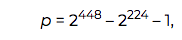Over a million developers have joined DZone.{{announcement.body}}
{{announcement.title}}

# Curve Ed448 and Karatsuba Multiplication

DZone 's Guide to

# Curve Ed448 and Karatsuba Multiplication

### Explore Curve Ed448 and Karatsuba multiplication.

· AI Zone ·
Free Resource

Comment (0)

Save
{{ articles.views | formatCount}} ViewsMike Hamburg designed an elliptic curve for use in cryptography he calls Ed448-Goldilocks. The prefix Ed refers to the fact that it's an Edwards curve. The number 448 refers to the fact that the curve is over a prime field where the prime p has size 448 bits. But why Goldilocks?

## Golden Primes and Goldilocks

The prime, in this case, iswhich has the same form as the NIST primes. Hamburg says in his paper:

I call this the "Goldilocks" prime because its form defines the golden ratio φ = 2 224.

That sentence puzzled me. What does this have to do with the golden ratio? The connection is that Hamburg's prime is of the form

φ² - φ - 1.

The roots of this polynomial are the golden ratio and its conjugate. But instead of looking for real numbers where the polynomial is zero, we're looking for integers where the polynomial takes on a prime value. (See the followup post on golden ratio primes.)

The particular prime that Hamburg uses is the "Goldilocks" prime by analogy with the fairy tale: the middle term 2 224 is just the right size. He explains

Because 224 = 32*7 = 28*8 = 56*4, this prime supports fast arithmetic in radix 2 28 or 2 32 (on 32-bit machines) or 2 56 (on 64-bit machines). With 16, 28-bit limbs it works well on vector units such as NEON. Furthermore, radix-2 64 implementations are possible with greater efficiency than most of the NIST primes.

## Karatsuba Multiplication

The title of this post is "Goldilocks and the three multiplications." Where do the three multiplications come in? It's an allusion to an algorithm for multi-precision multiplication that lets you get by with three multiplications where the most direct approach would require four. The algorithm is called Karatsuba multiplication .

Hamburg says "The main advantage of a golden-ratio prime is fast Karatsuba multiplication" and that if we set φ = 2 224 thenNote that the first line on the right side involves four multiplications, but the bottom line involves three. Since the variables represent 224-bit numbers, removing a multiplication at the expense of an extra addition and subtraction is a net savings .

The most important line of the calculation above, and the only one that isn't routine, is the second. That's where the special form of p comes in. When you eliminate common terms from both sides, the calculation boils down to showing thatwhich is obviously true since p = φ² - φ - 1.

## Curve Ed448-Goldilocks

Edwards curves only have one free parameter d (besides the choice of field) since they have the form

Hamburg chose d = -39081 for reasons explained in the paper.

Most elliptic curves used in ECC currently work over prime fields of order 256 bits, providing 128 bits of security. The motivation for developed Ed448 was much the same as for developing P-384. Both work over larger fields and so provide more bits of security, 224 and 192 bits respectively.

Unlike P-384, Ed448 is a safe curve, meaning that it lends itself to a secure practical implementation.

 Here we've just applied the Karatsuba algorithm one time. You could apply it recursively to multiply two n-bit numbers in O( n) time, where k = log 2 3 ≈ 1.86. This algorithm, discovered in 1960, was the first multiplication algorithm faster than O( n ²).

 Addition and subtraction are O( n) operations. And what about multiplication? That's not an easy question. It's no worse than O( n1.68) by virtue of the Karatsuba algorithm. In fact, it's O( n log n), but only for impractically large numbers. See the discussion here. But in any case, multiplication is slower than addition for multi-precision numbers.

Topics:
cryptography ,number theory ,ai ,curve ed448 ,karatsuba multiplication ,golden primes ,goldilocks principle

Comment (0)

Save
{{ articles.views | formatCount}} Views

Published at DZone with permission of

Opinions expressed by DZone contributors are their own.

# {{ parent.title || parent.header.title}}

{{ parent.tldr }}

{{ parent.urlSource.name }}